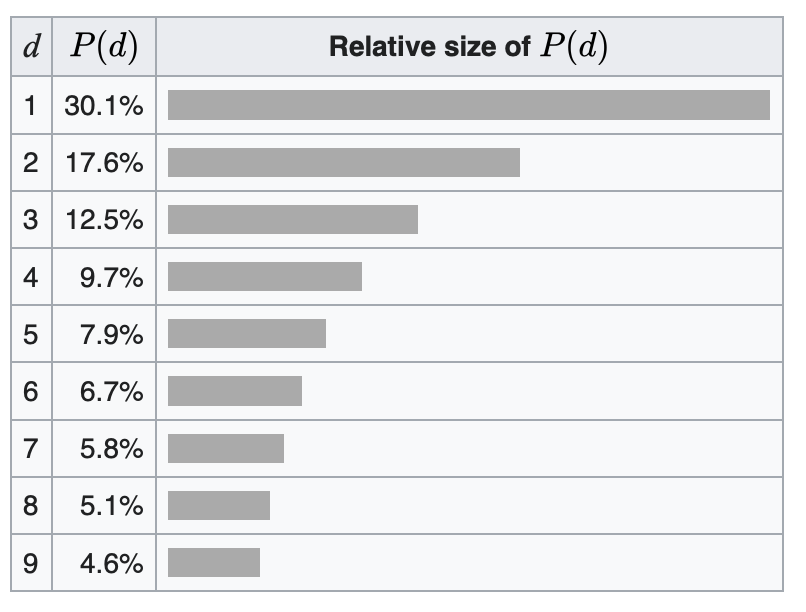# Benford's Law

Very similar to Zipf’s Law, which focused on the relationship of letters and words. Benford’s Law looks at the frequency of numbers starting with a particular number.

$P_{D}=\frac{\int_{D}^{D+1}P(x)dx}{\int_{1}^{10}P(x)dx}=log_{10}\left ( 1+\frac{1}{D} \right )$

Distribution:Written on January 10, 2023